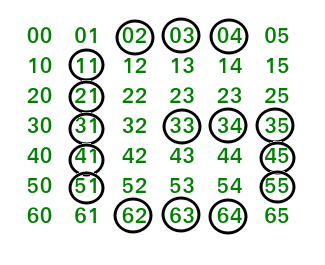GFG App
Open AppBrowser
Continue

# Python Program to print the pattern ‘G’

In this article, we will learn how to print the pattern G using stars and white-spaces. Given a number n, we will write a program to print the pattern G over n lines or rows.
Examples:

```Input : 7
Output :
***
*
*
* ***
*   *
*   *
***

Input : 9
Output :
*****
*
*
*
*   ***
*     *
*     *
*     *
*****  ```

In this program, we have used the simple logic of iteration over lines to create the pattern G. Please look at the image below which represents the pattern G in the form of a 2-d matrix, where mat[i][j] = ‘ij’:If we try to analyze this picture with a (row, column) matrix and the circles represent the position of stars in the pattern G, we will learn the steps. Here we are performing the operations column-wise. So for the first line of stars, we set the first if condition, where the row position with 0 and (n-1) won’t get the stars and all other rows from 1 to (n-1), will get the stars. Similarly, for the second, third and fourth column we want stars at the position row = 0 and row = (n-1). The other steps are self-explanatory and can be understood from the position of rows and columns in the diagram.
Below is the implementation of above idea:

## Python

 `# Python program to print pattern G` `def` `Pattern(line):` `    ``pat``=``""` `    ``for` `i ``in` `range``(``0``,line):    ` `        ``for` `j ``in` `range``(``0``,line):     ` `            ``if` `((j ``=``=` `1` `and` `i !``=` `0` `and` `i !``=` `line``-``1``) ``or` `((i ``=``=` `0` `or` `                ``i ``=``=` `line``-``1``) ``and` `j > ``1` `and` `j < line``-``2``) ``or` `(i ``=``=` `((line``-``1``)``/``2``)` `                ``and` `j > line``-``5` `and` `j < line``-``1``) ``or` `(j ``=``=` `line``-``2` `and` `                ``i !``=` `0` `and` `i !``=` `line``-``1` `and` `i >``=``((line``-``1``)``/``2``))):  ` `                ``pat``=``pat``+``"*"`   `            ``else``:      ` `                ``pat``=``pat``+``" "`   `        ``pat``=``pat``+``"` `"   ` `    ``return` `pat` ` `  `# Driver Code` `line ``=` `7` `print``(Pattern(line))`

## C++

 `// C++ program to print pattern G`   `#include ` `using` `namespace` `std;`   `string Pattern(``int` `line){` `    ``string pat = ``""``;` `    ``for``(``int` `i=0; i 1 and j < line-2) or (i == ((line-1)/2)` `                ``and j > line-5 and j < line-1) or (j == line-2 and` `                ``i != 0 and i != line-1 and i >=((line-1)/2))){` `                ``pat=pat+``"*"``;` `            ``} ``else``{` `                ``pat = pat + ``" "``;` `            ``}` `        ``pat = pat + ``"                                                                                                                                                              "``;` `        ``}` `    ``}` `    `  `    ``return` `pat;` `}`   `// Driver Code` `int` `main() {` `    ``int` `line = 7;` `    ``cout<

Output:

```  ***
*
*
* ***
*   *
*   *
***  ```

Time Complexity: O(n2), where n represents the given input.
Auxiliary Space: O(1), no extra space is required, so it is a constant.

Please refer complete article on Program to print the pattern ‘G’ for more details!

My Personal Notes arrow_drop_up